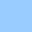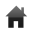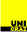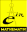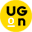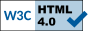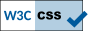# Numbers of isomorphism classes of binary and regular (connected) matroids

## Harald Fripertinger and Marcel Wild

In the following tables we list the numbers of non-isomorphic (k × n)-matrix matroids of rank (dimension) k.

If you are following the links you can download text-files in UNIX-format, which are compressed by gzip, containing complete sets of representatives of the corresponding matroids. The first line of each of these files contains the length n, the second line the rank k of the matroids in question. The letter 2 in the third line indicates that these are binary matroids. The fourth line shows the number of matroids contained in the file. Starting from the fifth line, each matrix matroid is represented by a sequence of n integer numbers written in one line. If you replace these integers by their binary representation and if you fill the columns of a matrix with these binary sequences, then you obtain the matrix matroid.

For instance, the simple binary matroids of length 4 and rank 3 are contained in the file `bin_simple4_3.txt`. It reads as follows.

```4
3
2
2
1 2 3 4
1 2 4 7
```

We see that these are matroids of length 4, rank 3, that these are binary matroids, and that there exist 2 non-isomorphic matroids. The binary representations of 1, 2, 3, 4, and 7 are 001, 010, 011, 100, and 111 respectively. Thus

```1 2 3 4    represents the matrix   1 0 1 0
0 1 1 0
0 0 0 1
```
and
```1 2 4 7    represents the matrix   1 0 0 1
0 1 0 1
0 0 1 1
```

harald.fripertinger "at" uni-graz.at, October 12, 2017